Next: Charged Conductors Up: Gauss' Law Previous: Electric Field of a

## Electric Field of a Uniformly Charged Plane

Consider an infinite plane which carries the uniform charge per unit area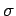. Suppose that the plane coincides with the-plane (i.e., the plane which satisfies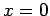). By symmetry, we expect the electric field on either side of the plane to be a function ofonly, to be directed normal to the plane, and to point away from/towards the plane depending on whetheris positive/negative.Let us draw a cylindrical gaussian surface, whose axis is normal to the plane, and which is cut in half by the plane--see Fig. 12. Let the cylinder run fromto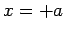, and let its cross-sectional area be. According to Gauss' law,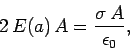(72)

where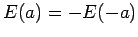is the electric field strength at. Here, the left-hand side represents the electric flux out of the surface. Note that the only contributions to this flux come from the flat surfaces at the two ends of the cylinder. The right-hand side represents the charge enclosed by the cylindrical surface, divided by. It follows that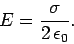(73)

Note that the electric field is uniform (i.e., it does not depend on), normal to the charged plane, and oppositely directed on either side of the plane. The electric field always points away from a positively charged plane, and vice versa.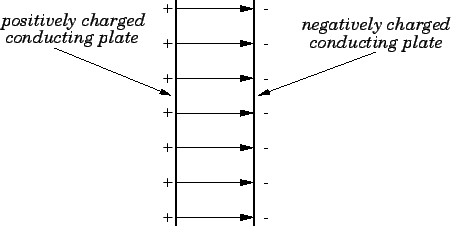Consider the electric field produced by two parallel planes which carry equal and opposite uniform charge densities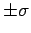. We can calculate this field by superposing the electric fields produced by each plane taken in isolation. It is easily seen, from the above discussion, that in the region between the planes the field is uniform, normal to the planes, directed from the positively to the negatively charged plane, and of magnitude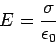(74)

--see Fig. 13. Outside this region, the electric field cancels to zero. The above result is only valid for two charged planes of infinite extent. However, the result is approximately valid for two charged planes of finite extent, provided that the spacing between the planes is small compared to their typical dimensions.Next: Charged Conductors Up: Gauss' Law Previous: Electric Field of a
Richard Fitzpatrick 2007-07-14